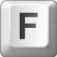fairy hunter - 2 years ago 116
Java Question

# Introduction

I have an infinite String. The length of this string is infinite in our imagination and cannot be limited.Suppose that we have a sequence String like this:

"123456789..."

The points after the number 9 actually representing the next sequence. So, it will be like this:

"...7891011121314..."

# Requirements

In this section, I want to explain about the requirement. The requirement is to find the index of first occurrence of input String (called n). Let me give you an example:

## Example 1

n="3"

First Index of n = 2

## Example 2

n="910"

First Index of n = 8

# Problems

I have coded the algorithm to find the index of the String n. But the algorithm is only just a while loop to check the index of n and add the next sequence number one by one if index of n is not found. I want a better algorithm for this to find the index of first occurrence of n without relying on looping or less looping. At least, the algorithm don't run longer than 2 seconds if the value of n is big (Example: 123456790 or 62716855).

### ---Edit---

Snippet of my Code:

``````while(!num.contains(s)){
num +=start.toString();
}
``````

This is my full code: Full Code of My CodeEran

Here's a general description of how to solve this problem. Translating it to Java can still be challenging.

Your input String is basically an infinite sequence of all the natural numbers 1 2 3 4 5 6 8 9 10 11 ....

I believe the point of the exercise is to identify the first sub-sequence of natural numbers of the input String that the substring `n` belongs to, and then calculate its index without actually constructing the large "infinite" String.

For that purpose, you have to try to split the substring `n` into an incrementing sequence of numbers having as few digits as possible.

First you have to check is the substring `n` creates a sequence of single digits numbers. That is the case, for example, if n == 345678 (note that n may contain both single digit and double digit numbers, for example `n == 345678910`, which you should also be able to identify).

If you fail in that step, you should look for a sequence of double digit numbers. That is the case, for example, if `n == 33343536`. Now, this could get trickier, since `n == 2333435363` is also a sequence of two digit numbers, but the leading and trailing numbers of the sequence (32 and 37) are truncated.

If you fail again, you look for a sequence of 3 digit numbers.

If you don't find any sequence, you treat the entire substring `n` as a single number in the big String.

Now, suppose `n` is `199319941995`, and you discovered in the previous step that the first number in the sequence is `1993`. The remaining work is to calculate the index of the number `1993` in the input String. You know that the single digit numbers take 1*9 indices. The two digit numbers take 2*90 indices. The three digit numbers take 3*900 indices. The 3 digit numbers between 1000 and 1993 take 4*993 indices. Therefore the index of 1993 is 1*9+2*90+3*900+4*993, and that's the first index of the substring `199319941995`.

Recommended from our users: Dynamic Network Monitoring from WhatsUp Gold from IPSwitch. Free Download